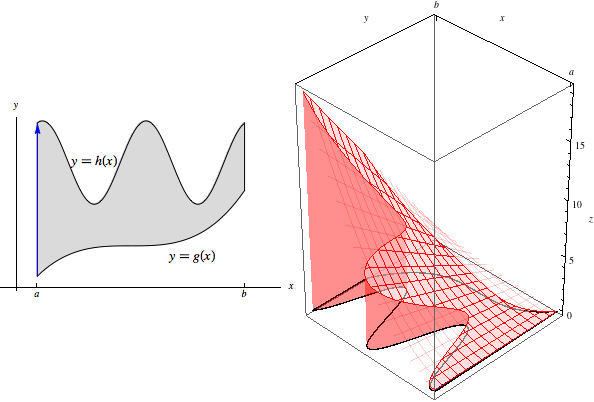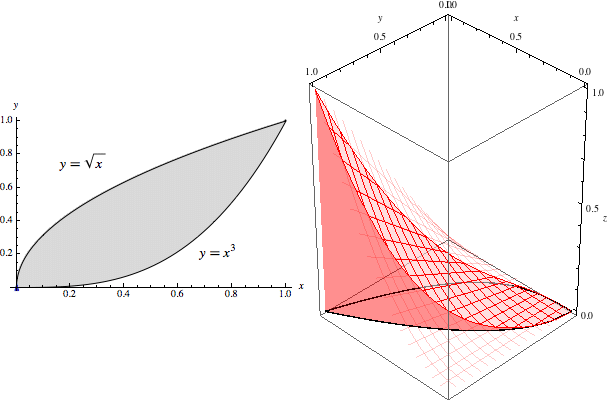# Math 215 Examples

## Double Integrals over General Regions

### Key Concepts

#### Regions of Type I and II

A region in the $$xy$$-plane is called type I if it is defined as the region between two functions of $$x$$:A region in the $$xy$$-plane is called type II if it is defined as the region between two functions of $$y$$:#### Integrating over Regions of Type I

Let $$R$$ be the type I region lying between $$y=g(x)$$ and $$y=h(x)$$ for $$a\le x\le b$$. Then

$\iint\limits_R f(x,y)\, dA = \int_a^b\int_{g(x)}^{h(x)} f(x,y)\, dy\, dx.$

To visualize this, imagine the slice at a fixed value of $$x$$. This slice begins at $$g(x)$$ and ends at $$h(x)$$. Shown below-left is the region in the $$xy$$-plane, with the slice drawn as a blue arrow, and below-right is the slice drawn in a 3D-coordinate system. (Note that the origin in the 3D-coordinate system is at the bottom right of the box.)The area under this slice is $\text{Area}(x)=\int_{g(x)}^{h(x)} f(x,y)\, dy.$ As with rectangular regions, then, to compute the original double integral, we sweep through the entire volume as these slices range from $$x=a$$ to $$x=b$$:This yields the final result of $\iint\limits_R f(x,y)\, dA = \int_a^b \text{Area}(x)\, dx = \int_a^b\int_{g(x)}^{h(x)} f(x,y)\, dy\, dx.$

#### Integrating over Regions of Type II

Let $$R$$ be the type II region lying between $$x=g(y)$$ and $$x=h(y)$$ for $$a\le y\le b$$. Then

$\iint\limits_R f(x,y)\, dA = \int_a^b\int_{g(y)}^{h(y)} f(x,y)\, dx\, dy.$

This situation is analogous to the one above for type I regions, but now our slices are at fixed $$y$$-values. The slice at $$y$$ begins at $$g(y)$$ and ends at $$h(y)$$:The area under this slice is $\text{Area}(y)=\int_{g(y)}^{h(y)} f(x,y)\, dx.$ As these slices of area range through from $$y=a$$ to $$y=b$$, we sweep through the entire volume:Hence $\iint\limits_R f(x,y)\, dA = \int_a^b \text{Area}(y)\, dy = \int_a^b\int_{g(y)}^{h(y)} f(x,y)\, dx\, dy.$

### Illustrated Example

Example Let $$R$$ be the region in the $$xy$$-plane bounded by the curves $$y=x^3$$ and $$y=\sqrt{x}$$. Evaluate $\iint\limits_R x^2 y\, dA.$

#### Worked Solution

Let us first look at this region $$R$$ in the $$xy$$-plane:The intersection points occur when $$x^3=\sqrt{x}$$, which we can solve to find $$x=0$$ and $$x=1$$. Notice that for $$0\le x\le 1$$, the curve $$y=\sqrt{x}$$ is on top and $$y=x^3$$ is on the bottom. Hence $$R$$ is the type I region defined by $$0\le x\le 1$$ and $$x^3\le y\le \sqrt{x}$$.

We can now write the desired double integral as an iterated integral: $\iint\limits_R x^2 y\, dA = \int_0^1\int_{x^3}^{\sqrt{x}} x^2 y\, dy\, dx$ We evaluate the inner integral treating $$x$$ as constant: \begin{aligned}\int_{x^3}^{\sqrt{x}} x^2 y\, dy &= \frac{1}{2}x^2y^2 \Big|_{x^3}^{\sqrt{x}} \\ &= \frac{1}{2}(x^3-x^8) \end{aligned}

Using this, we have \begin{aligned} \iint\limits_R x^2 y\, dA &= \int_0^1\int_{x^3}^{\sqrt{x}} x^2 y\, dy\, dx \\ &= \int_0^1 \frac{1}{2}(x^3-x^8)\, dx \\ &= (x^4/8 - x^9/18) \Big|_0^1 \\ &= (1/8-1/18)-(0-0) = 5/72. \end{aligned}

#### Visualizing the Example

Our inner integral $$\int_{x^3}^{\sqrt{x}} x^2y\, dy$$ gives the area of the slice at any $$x$$ value. For example, at $$x=0.6$$, we have the slice shown below.Plugging $$x=0.6$$ into this integral, we see that the area of this slice is \begin{aligned} \int_{0.6^3}^{\sqrt{0.6}} 0.6^2y\, dy &= \int_{0.216}^{\sqrt{0.6}} 0.36y\, dy \\ &= 0.18y^2\Big|_{0.216}^{\sqrt{0.6}} \\ &= 0.18(0.6 - 0.216^2) = 0.0996. \end{aligned} Note that this is exactly what we get when we plug $$x=0.6$$ into $$\frac{1}{2}(x^3-x^8)$$, as we computed in general that $\int_{x^3}^{\sqrt{x}} x^2y\, dy = \frac{1}{2}(x^3-x^8).$

As we let $$x$$ range from $$0$$ to $$1$$, these slices sweep out the entire volume of $$5/72$$:#### Further Questions

1. What is the area under the slice at $$x=0.4$$? What is the area under the slice at $$x=1$$?
2. Suppose we switched the bounds on the inner integral and instead integrated from $$\sqrt{x}$$ to $$x^3$$. How would this mistake affect the final answer?
3. The region $$R$$ in the example is also a type II region. What functions $$x=g(y)$$ and $$x=h(y)$$ bound it?
4. Evaluate the integral in the example by treating $$R$$ as the type II region you described in Question 3.

### Using the Mathematica Demo

All graphics on this page were generated by the Mathematica notebook 15_3DoubleIntegralsOverGeneralRegions.

This notebook generates images and animations like those on this page for any type I region with $$y=g(x)$$ and $$y=h(x)$$. The notebook also provides many additional options, including the option to attempt to treat the region as type II.

As an exercise, use the notebook to provide a clear graphical answer to Question 3. You can do this simply by setting regionType = 2 and re-executing the code in the Graphics section. Then feel free to change the bounds and the function $$f(x,y)$$ itself, keeping in mind the limitations described in the notebook.